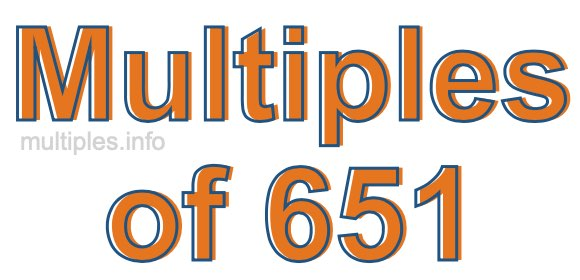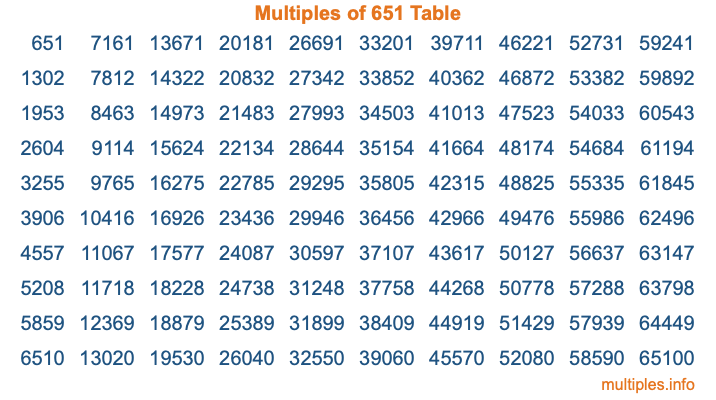Multiples of 651Welcome to the Multiples of 651 page. Here we will first teach you everything you will ever need to know about the multiples of 651, and then give you a study guide summary of everything we taught you to make sure you remember it all. Use this page to look up facts and learn information about the multiples of 651. This page will make you a multiples of six hundred fifty-one expert!

Definition of Multiples of 651
Multiples of 651 are all the numbers that when divided by 651 equal an integer. Each of the multiples of 651 are called a multiple. A multiple of 651 is created by multiplying 651 by an integer.

Therefore, to create a list of multiples of 651, you start with 1 multiplied by 651, then 2 multiplied by 651, then 3 multiplied by 651, and so on for as long as you want. Thus, the list of the first five multiples of 651 is 651, 1302, 1953, 2604, and 3255. To see a larger list of multiples of 651, see the printable image of Multiples of 651 further down on this page. We also have a category where you can choose any nth multiple of 651.

Multiples of 651 Checker
The Multiples of 651 Checker below checks to see if any number of your choice is a multiple of 651. In other words, it checks to see if there is any number (integer) that when multiplied by 651 will equal your number. To do that, we divide your number by 651. If the the quotient is an integer, then your number is a multiple of 651.

Is  a multiple of 651?

Least Common Multiple of 651 and ...
A Least Common Multiple (LCM) is the lowest multiple that two or more numbers have in common. This is also called the smallest common multiple or lowest common multiple and is useful to know when you are adding our subtracting fractions. Enter one or more numbers below (651 is already entered) to find the LCM.

Check out our LCM Calculator if you need more details about the Least Common Multiple or if you need the LCM for different numbers for adding and subtraction fractions.

nth Multiple of 651
As we stated above, 651 is the first multiple of 651, 1302 is the second multiple of 651, 1953 is the third multiple of 651, and so on. Enter a number below to find the nth multiple of 651.

th multiple of 651

Multiples of 651 vs Factors of 651
651 is a multiple of 651 and a factor of 651, but that is where the similarities end. All postive multiples of 651 are 651 or greater than 651. All positive factors of 651 are 651 or less than 651.

Below is the beginning list of multiples of 651 and the factors of 651 so you can compare:

Multiples of 651: 651, 1302, 1953, 2604, 3255, etc.

Factors of 651: 1, 3, 7, 21, 31, 93, 217, 651

As you can see, the multiples of 651 are all the numbers that you can divide by 651 to get a whole number. The factors of 651, on the other hand, are all the whole numbers that you can multiply by another whole number to get 651.

It's also interesting to note that if a number (x) is a factor of 651, then 651 will also be a multiple of that number (x).

Multiples of 651 vs Divisors of 651
The divisors of 651 are all the integers that 651 can be divided by evenly. Below is a list of the divisors of 651.

Divisors of 651: 1, 3, 7, 21, 31, 93, 217, 651

The interesting thing to note here is that if you take any multiple of 651 and divide it by a divisor of 651, you will see that the quotient is an integer.

Multiples of 651 Table
Below is an image of the first 100 multiples of 651 in a table. The table is in chronological order, column by column. The first column has the first ten multiples of 651, the second column has the next ten multiples of 651, and so on.The Multiples of 651 Table is also referred to as the 651 Times Table or Times Table of 651. You are welcome to print out our table for your studies.

Negative Multiples of 651
Although not often discussed or needed in math, it is worth mentioning that you can make a list of negative multiples of 651 by multiplying 651 by -1, then by -2, then by -3, and so on, to get the following list of negative multiples of 651:

-651, -1302, -1953, -2604, -3255, etc.

Multiples of 651 Summary
Below is a summary of important Multiples of 651 facts that we have discussed on this page. To retain the knowledge on this page, we recommend that you read through the summary and explain to yourself or a study partner why they hold true.

There are an infinite number of multiples of 651.

A multiple of 651 divided by 651 will equal a whole number.

651 divided by a factor of 651 equals a divisor of 651.

The nth multiple of 651 is n times 651.

The largest factor of 651 is equal to the first positive multiple of 651.

651 is a multiple of every factor of 651.

651 is a multiple of 651.

A multiple of 651 divided by a divisor of 651 equals an integer.

651 divided by a divisor of 651 equals a factor of 651.

Any integer times 651 will equal a multiple of 651.

Multiples of a Number
Here you can get the multiples of another number, all with the same attention to detail as we did for multiples of 651 on this page.

Multiples of
Multiples of 652
Did you find our page about multiples of six hundred fifty-one educational? Do you want more knowledge? Check out the multiples of the next number on our list!